# 【Python】Python基础知识（一）：数据类型，格式化输出，运算符

Debug工具

f-格式化字符串

Python解释器和PyCharm下载与安装

## 注释

# 这是单行注释
'''

'''
"""

"""
print("Hello")  # 这是单行注释

## 变量

# 定义变量
strText = "haha"
# 使用变量
print(strText)

## Debug工具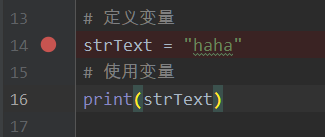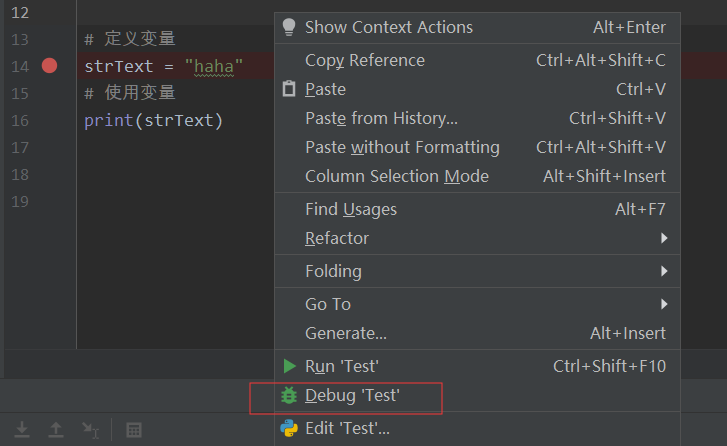## 数据类型

num1 = 1
num2 = 1.1

print(type(num1))  # int
print(type(num2))  # float

b = False
print(type(b))  # bool

strText = "Hello"
print(type(strText))  # str

tempList = [1, 2, 3]
print(type(tempList))  # list

tempTuple = (1, 2, 3)
print(type(tempTuple))  # tuple

tempSet = {1, 2, 3}
print(type(tempSet))  # set

tempDict = {"name": "haha", "age": 18}
print(type(tempDict))  # dict

print(type(type))  # type

print(type(print))  # builtin_function_or_method

## 格式化输出

%s字符串
%d有符号的十进制整数
%f浮点数
%c字符
%u无符号十进制整数
%o八进制整数
%x十六进制整数（小写0x）
%X十六进制整数（大写0X）
%e科学计数法（小写“e”）
%E科学计数法（大写“E”）
%g%f和%e的简写
%G%f和%E的简写

name = "哈哈"
age = 18
weight = 50.5
id = 1

print("我是%s" % name)
print("我今年%d岁" % age)

print("我的体重是%f公斤" % weight)  # 输出结果 我的体重是50.500000公斤
print("我的体重是%.2f公斤" % weight)  # 保留两位小数

print("我的学号是%d" % id)
print("我的学号是%03d" % id)  # 格式化输出3位
print("我的学号是%03d" % 10)  # 格式化输出3位

print("我的名字是%s，今年%d岁了" % (name, age))   # 输出多个变量

### f-格式化字符串

（Python3.6新增格式化方法）

print("我的名字是%s，今年%d岁了" % (name, age))
print(f"我的名字是{name}，今年{age}岁了")

## 转义字符

\n ：换行（反斜杠）

\t ：制表符，一个tab键（4个空格）的距离

\n使用

print("Hello")  # 不用\n换行
print("World")

print("Hello\nWorld")  # 用\n换行

\t使用

print("haha")
print("\thaha")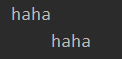## 结束符

print("Hello")  # 不用\n换行
print("World")

# 默认自带end="\n"

print("Hello", end="")
print("World")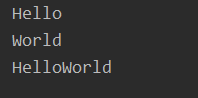## 输入

str = input("请输入你的密码：")
print(str)## 数据类型转换

int(x,[base])将x转换为一个整数
float(x)将x转换为一个浮点数
str(x)将x转换为一个字符串
eval(str)用来计算在字符串中的有效Python表达式，并返回一个对象
tuple(s)将序列s转换为一个元组
list(s)将序列s转换为一个列表

strNum = input("请输入数字：")
num = int(strNum)

eval()

str1 = "1"
str2 = "1.1"
print(type(eval(str1)))
print(type(eval(str2)))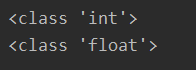## 运算符

+1+1结果为2
-1-1结果为0
*2*3结果为6
/9/2结果为4.5
//整除9//2结果为4
%取余9%2结果为1
**指数2**3结果为8，即2*2*2
()小括号提高运算优先级

 运算符 描述 例子 = 赋值 将=右侧的结果赋值给等号左侧的变量

num1 = 1

num1, float1, str1 = 1, 1.1, "haha"

num1 = num2 = num3 = 10

+=加法赋值运算符s += a 等价于 s = s + a
-=减法赋值运算符s -= a 等价于 s = s - a
*=乘法赋值运算符s *= a 等价于 s = s * a
/=除法赋值运算符s /= a 等价于 s = s / a
//=整除赋值运算符s //= a 等价于 s = s // a
%=取余赋值运算符s %= a 等价于 s = s % a
**=幂赋值运算符s **= a 等价于 s = s ** a

# 先算复合赋值运算符右边的
s = 2
s *= 10 + 3
print(s)  # 26

==等于
!=不等于
>大于
<小于
>=大于等于
<=小于等于

 运算符 逻辑表达式 描述 and x and y 与 or x or y 或 not not x 非

and 运算符，只要有一个值为0，则结果为0，否则结果最后一个非0数字

or 运算符，只有所有值为0结果才为0，否则结果为第一个非0数字

print(0 and 2)  # 0
print(1 and 0)  # 0
print(1 and 2)  # 2
print(2 and 1)  # 1

print(0 or 0)  # 0
print(1 or 0)  # 1
print(0 or 2)  # 2
print(1 or 2)  # 1
print(2 or 1)  # 2

### 下篇：Python基础知识（二）：if语句，while循环，for循环

01-2956801-23639
12-0824
07-28669
08-2538
12-1520
04-1552
01-091156
04-2222
06-293075
03-11
09-042217
11-158915
02-1028
03-06786
07-31191
08-20707
04-14467
©️2020 CSDN 皮肤主题: 精致技术 设计师:CSDN官方博客